Скачать презентацию Decision Trees Chapter 18 From Data to Knowledge

f81fe635ca14a4811516282f40ff8fd5.ppt

• Количество слайдов: 17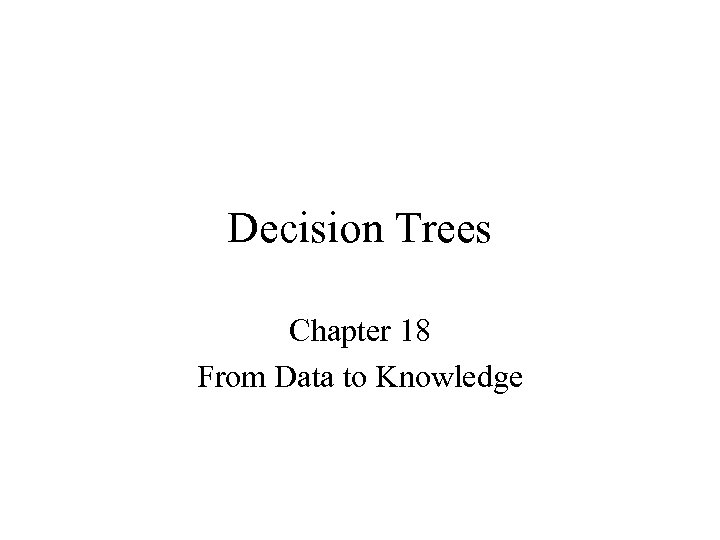Decision Trees Chapter 18 From Data to Knowledge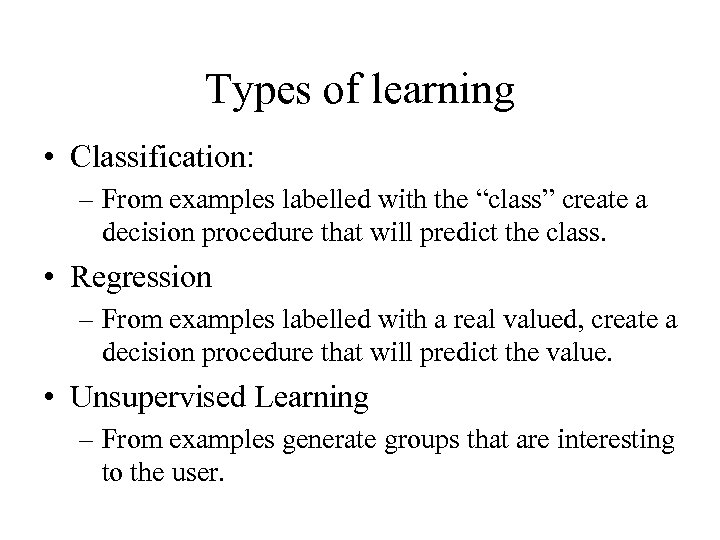Types of learning • Classification: – From examples labelled with the “class” create a decision procedure that will predict the class. • Regression – From examples labelled with a real valued, create a decision procedure that will predict the value. • Unsupervised Learning – From examples generate groups that are interesting to the user.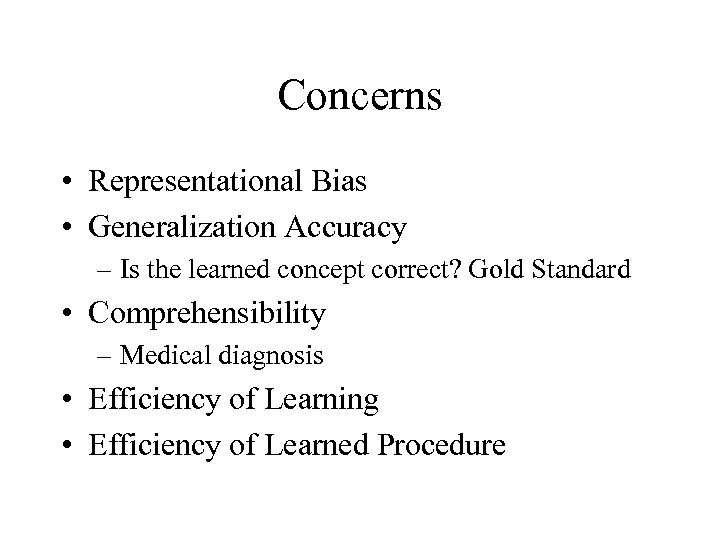Concerns • Representational Bias • Generalization Accuracy – Is the learned concept correct? Gold Standard • Comprehensibility – Medical diagnosis • Efficiency of Learning • Efficiency of Learned Procedure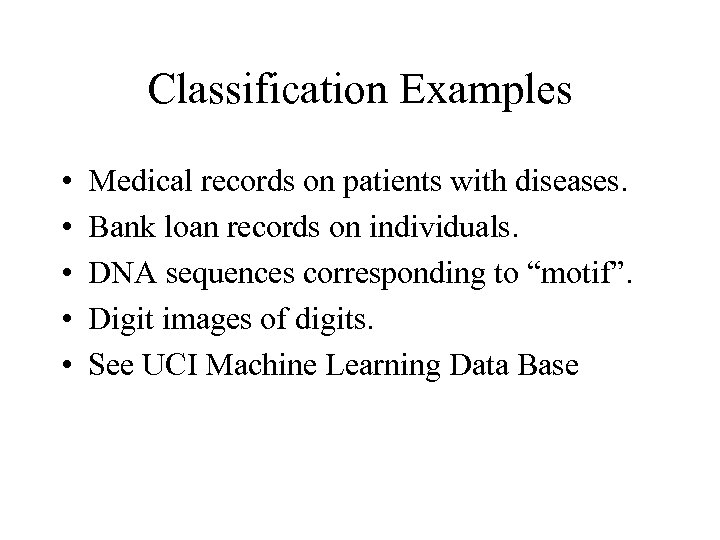Classification Examples • • • Medical records on patients with diseases. Bank loan records on individuals. DNA sequences corresponding to “motif”. Digit images of digits. See UCI Machine Learning Data Base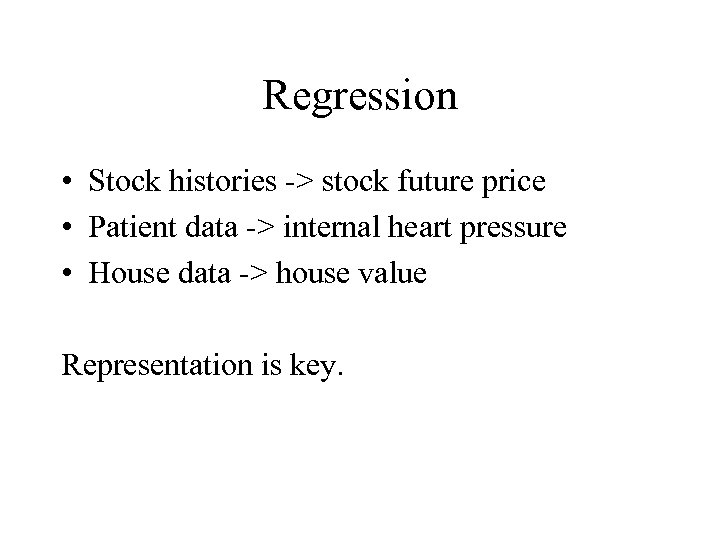Regression • Stock histories -> stock future price • Patient data -> internal heart pressure • House data -> house value Representation is key.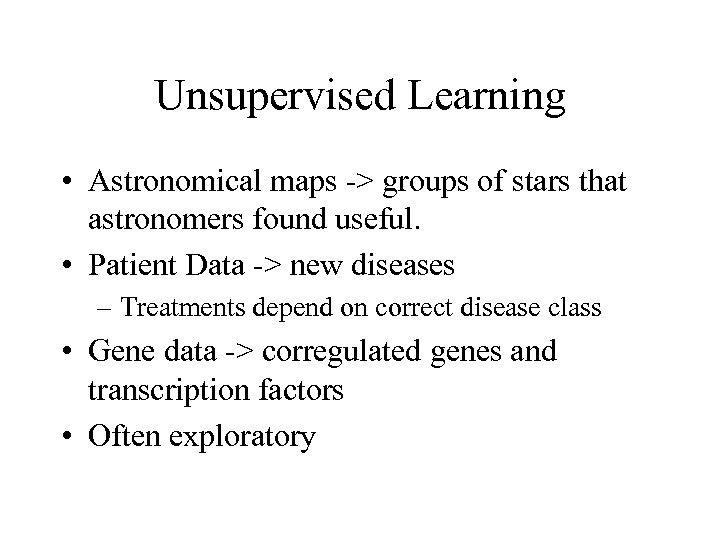Unsupervised Learning • Astronomical maps -> groups of stars that astronomers found useful. • Patient Data -> new diseases – Treatments depend on correct disease class • Gene data -> corregulated genes and transcription factors • Often exploratory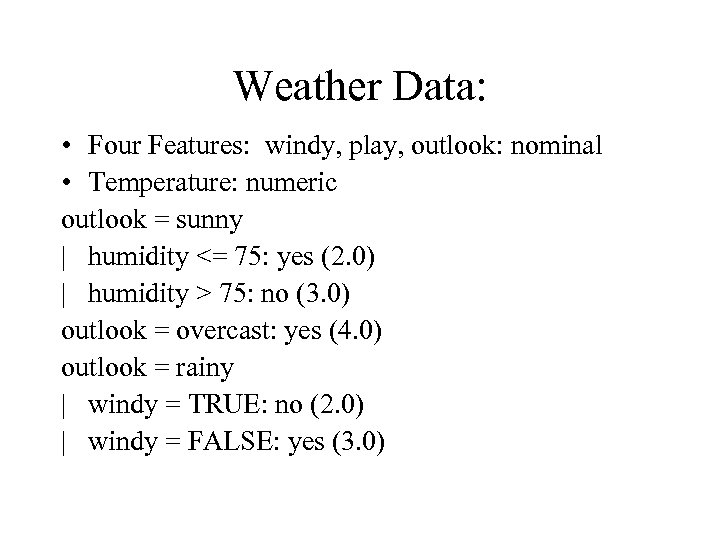Weather Data: • Four Features: windy, play, outlook: nominal • Temperature: numeric outlook = sunny | humidity <= 75: yes (2. 0) | humidity > 75: no (3. 0) outlook = overcast: yes (4. 0) outlook = rainy | windy = TRUE: no (2. 0) | windy = FALSE: yes (3. 0)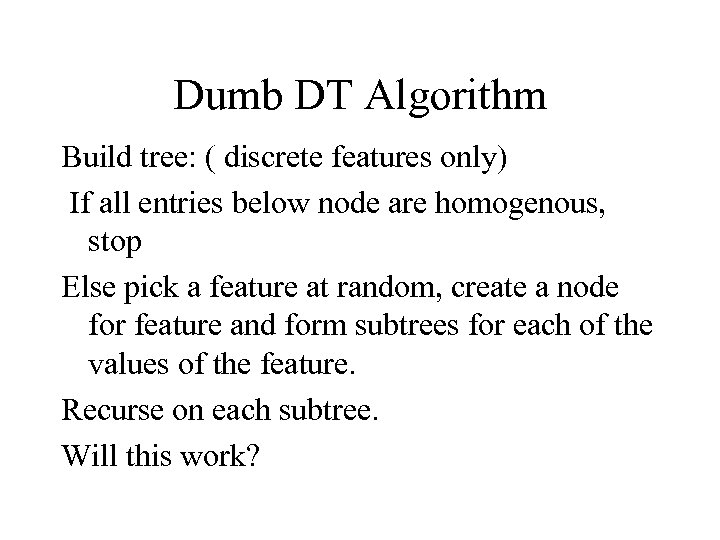Dumb DT Algorithm Build tree: ( discrete features only) If all entries below node are homogenous, stop Else pick a feature at random, create a node for feature and form subtrees for each of the values of the feature. Recurse on each subtree. Will this work?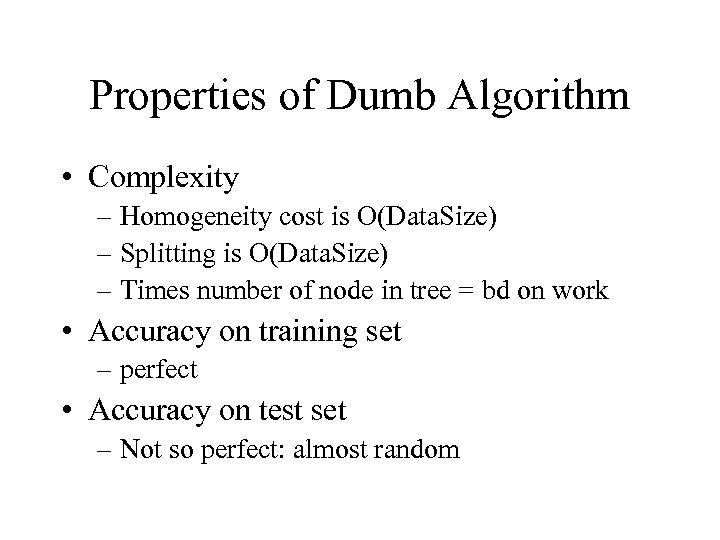Properties of Dumb Algorithm • Complexity – Homogeneity cost is O(Data. Size) – Splitting is O(Data. Size) – Times number of node in tree = bd on work • Accuracy on training set – perfect • Accuracy on test set – Not so perfect: almost random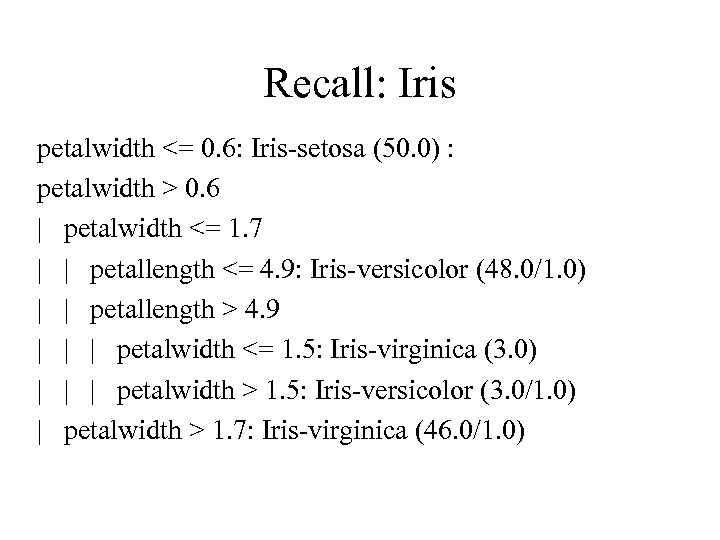Recall: Iris petalwidth <= 0. 6: Iris-setosa (50. 0) : petalwidth > 0. 6 | petalwidth <= 1. 7 | | petallength <= 4. 9: Iris-versicolor (48. 0/1. 0) | | petallength > 4. 9 | | | petalwidth <= 1. 5: Iris-virginica (3. 0) | | | petalwidth > 1. 5: Iris-versicolor (3. 0/1. 0) | petalwidth > 1. 7: Iris-virginica (46. 0/1. 0)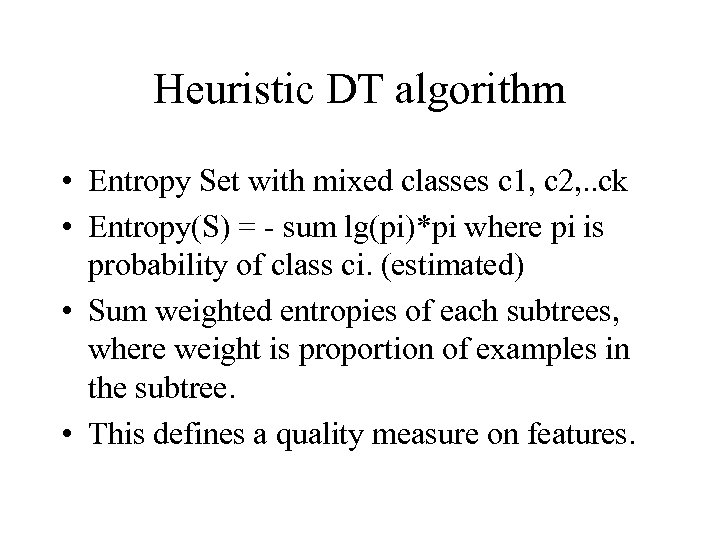Heuristic DT algorithm • Entropy Set with mixed classes c 1, c 2, . . ck • Entropy(S) = - sum lg(pi)*pi where pi is probability of class ci. (estimated) • Sum weighted entropies of each subtrees, where weight is proportion of examples in the subtree. • This defines a quality measure on features.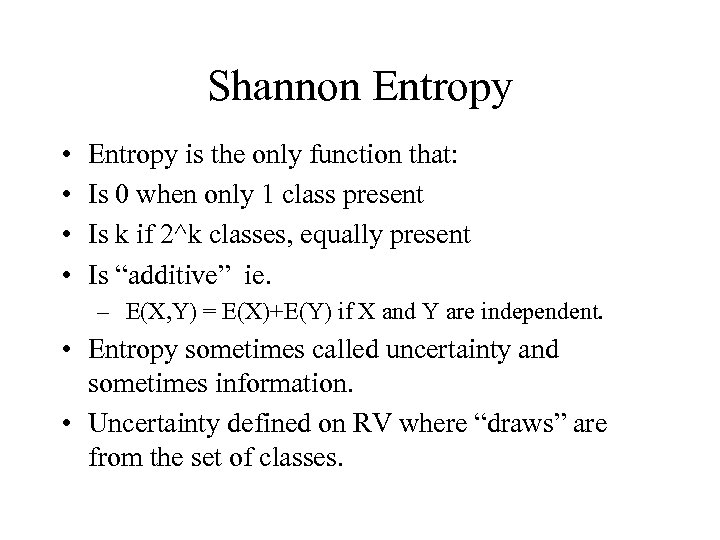Shannon Entropy • • Entropy is the only function that: Is 0 when only 1 class present Is k if 2^k classes, equally present Is “additive” ie. – E(X, Y) = E(X)+E(Y) if X and Y are independent. • Entropy sometimes called uncertainty and sometimes information. • Uncertainty defined on RV where “draws” are from the set of classes.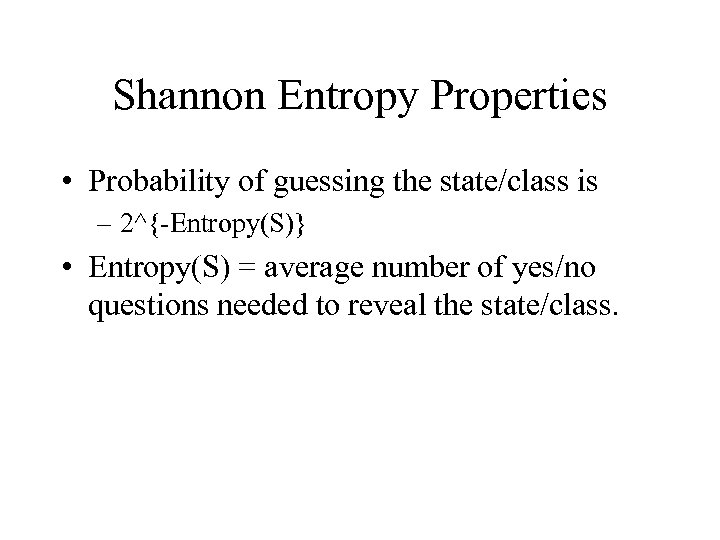Shannon Entropy Properties • Probability of guessing the state/class is – 2^{-Entropy(S)} • Entropy(S) = average number of yes/no questions needed to reveal the state/class.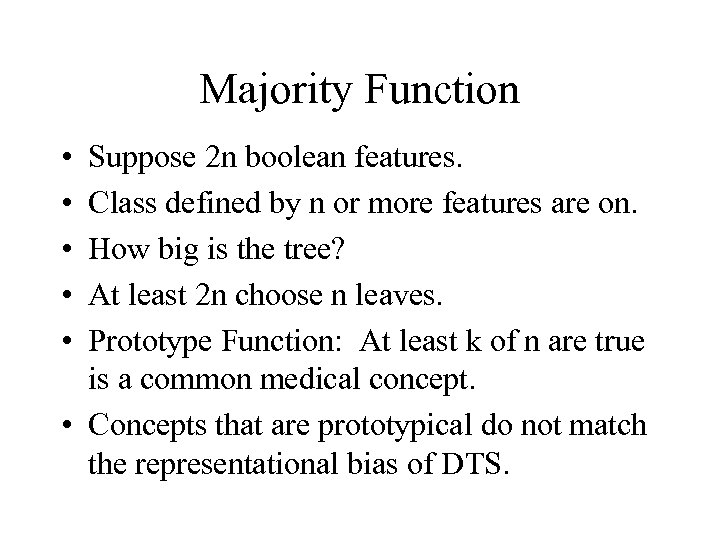Majority Function • • • Suppose 2 n boolean features. Class defined by n or more features are on. How big is the tree? At least 2 n choose n leaves. Prototype Function: At least k of n are true is a common medical concept. • Concepts that are prototypical do not match the representational bias of DTS.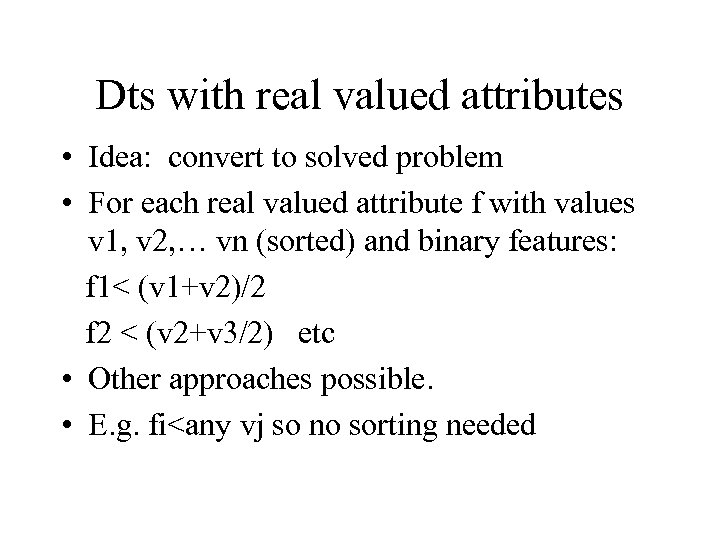Dts with real valued attributes • Idea: convert to solved problem • For each real valued attribute f with values v 1, v 2, … vn (sorted) and binary features: f 1< (v 1+v 2)/2 f 2 < (v 2+v 3/2) etc • Other approaches possible. • E. g. fi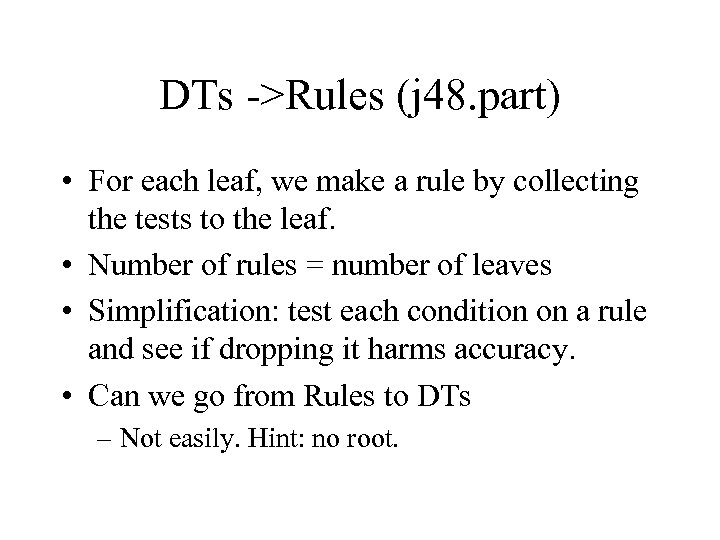DTs ->Rules (j 48. part) • For each leaf, we make a rule by collecting the tests to the leaf. • Number of rules = number of leaves • Simplification: test each condition on a rule and see if dropping it harms accuracy. • Can we go from Rules to DTs – Not easily. Hint: no root.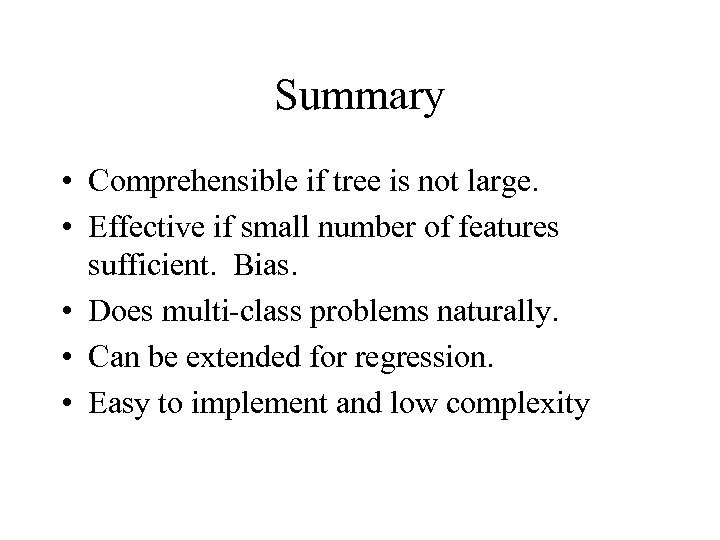Summary • Comprehensible if tree is not large. • Effective if small number of features sufficient. Bias. • Does multi-class problems naturally. • Can be extended for regression. • Easy to implement and low complexity